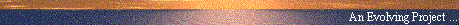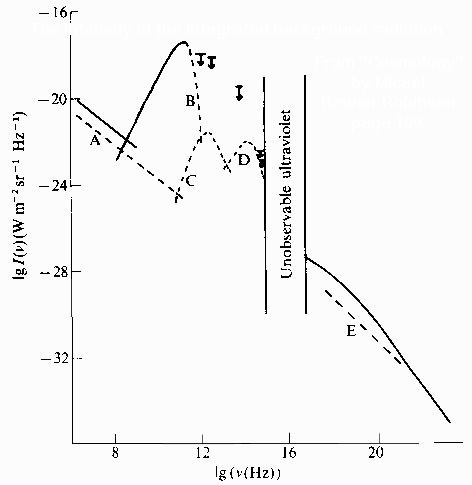# Photon counts ...

#### A post by Ray Tomes

##### Web Publication by Mountain Man Graphics, Australia - Southern Autumn '97## The Cosmic Distribution of Photons

Date: Fri, 02 May 1997 01:36:14 GMT
From: rtomes@kcbbs.gen.nz (Ray Tomes)
Organization: KC Computer Services
Newsgroups: sci.physics, sci.physics.relativity, sci.astro, alt.sci.physics.new-theories
Subject: Photon counts for Mountain Man (was Re: why the aether?)

Mountain Man in article <33688655.41E5@magna.com.au> wrote:

Can you or anyone else point me in the direction of resources
which would enable me to tabulate this CMBR "photon density"
according to different sections (wavelength/frequency) of the EM spectrum?

Ray Tomes replied ...There is a graph on my WWW site at which shows the log of the flux in Watts per square metre per Hz per steradian as a function of log frequency in intergalactic space. The solid line shows measured flux and the dashed line estimated flux. The graph covers the range of frequencies 10^6 to 10^23 Hz and shows a clear trend (a slope of nearly -1) except for three bumps, A=CMBR, B=infrared from dust, C=light from galaxies/stars.

To convert the above measurements to photons per cm^3 requires a number of steps. I think that the following is correct (someone please check)

1. Multiply by 4pi to get the total flux over all directions (from steradians).

2. Convert 1 Watt --> 10^7 erg/s.

3. Use E=hf (where h=6.626*10^-27 erg-s) to calculate the energy of one photon at any frequency and divide into your previous answer. [Of course you know my view that there is no such thing as a photon in flight, but this is how many photons could be absorbed from that much energy].

4. A square metre by a second needs to be multiplied by (100 cm/m)^2*c to get a volume in cm^3.

You then have the answer as No. of photons per cubic centimetre per Hz. Remember that there are a lot more Hz at high frequencies than at low ones. I prefer to look at things as energy density per order of magnitude of frequency which is almost flat apart from the 3 bumps mentioned.

To recap, take plotted figures *4pi /hf *(100cm/m)^2/c.

So Log(Photon density[/cm^3]) = Log(Flux[Watts per square metre per Hz per steradian]) - Log(f[Hz]) + Log(4pi*100^2/c[cm/s]/h[erg.s]) where that constant comes out to 20.8 (i.e. Log(6.32*10^20)). All Logs in base 10.

These are some figures read roughly off the graph and some calculated data:

```
background  <---  peaks  --->   background
CMB   IR   Light

Log(f[Hz])             6     8    11    12.5   14    17    20     23

Log(Flux)            -19.5 -21.5 -17.5 -21.5  -22   -27   -30.5  -35

Log(Photons/cm^3/Hz)  -4.7  -8.7  -7.7 -13.2  -15.2 -23.2 -29.7  -37.2

Log(Photons)
/Log(f[Hz])            1.3  -0.7   3.3  -0.7   -1.2  -6.2  -9.7  -14.2

```

The bottom line may be interpreted as follows... The 1.3 figure means that there are about 10^1.3 = 20 photons per 1 change in log(f) around 10^6 Hz (say between 10^5.5 and 10^6.5 Hz). The 3.3 figure gives 10^3.3 or ~3000 photons in the CMBR which is too high because the figure is a paek and so is not so high over a whole order of magnitude of frequency. At the other end, there are ~10-^14.2 = 6*10^-15 photons per cm^3 of around 10^23 Hz or just 6 photons per cubic Km.

When Cosmologists say that the total is 400 photons per cm^3 they are only including the CMBR and the higher frequencies (which are negligable in photon count). This is a gross error.

If the part of the spectrum at low (unmeasured) frequencies is extrapolated with the trend of the background of this graph then it is found that the photon count per cm^3 per order of magnitude frequency would be about 10^7 at 1 Hz, 10^12 at 10^-6 Hz, 10^17.5 at 10^-12 Hz and 10^21 at 10^-17 Hz. In other words there are most likely over 1,000,000,000,000,000,000,000 photons per cm^3 in the universe.

In a debate on this subject with Ted Bunn (a Cosmologist) a year or so ago I suggested that something like this was the case. He said that, no, the energy dropped off beyond the CMBR and eventually I found the above mentioned graph in a cosmology book. Ted now acknowledges that the graph does turn up again at frequencies below the CMBR but expects it to turn down just beyond the end of the graph because that is what known physics indicates. I reckon that known physics would have made the same mistake at every stage up the graph and shouldn't be trusted, but that the trend of the graph should be trusted.

I also believe that it is this blind spot for cosmologists (not just Ted) that fails to recognise that the low frequency e/m radiation can and does explain the missing mass.

### Ray Tomes

E-Mail: rtomes@kcbbs.gen.nz

WWW: Harmonics Theory -- http://www.kcbbs.gen.nz/users/rtomes/rt-home.htm# Photon counts ...

#### A post by Ray Tomes

##### Web Publication by Mountain Man Graphics, Australia - Southern Autumn '97